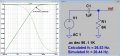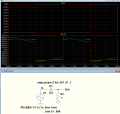# convert 0 to 5V square to square wave centered at 0V

#### cktboy

Joined Apr 24, 2020
45
Hello,
I have 0V to 5V square wave output from 555 timer. How to convert it with center at 0V, that is from -2.5V to 2.5V. I tried capacitor but I am getting -5V to 0V.

#### AlbertHall

Joined Jun 4, 2014
12,267
Welcome to AAC, @cktboy
What is the mark/space ratio and is it fixed or variable?

•cktboy

#### cktboy

Joined Apr 24, 2020
45
It is a square wave so the mark space ratio is 1:1 and it is fixed.

#### ericgibbs

Joined Jan 29, 2010
17,993
Hi cb,
What is the frequency and what is the output driving into.?
E

#### cktboy

Joined Apr 24, 2020
45
The frequency is 100khz and it is not driving into anything. I just wanted to learn how to level shift the output with center at 0V.

#### cktboy

Joined Apr 24, 2020
45
hi cb,
This R/C shows approx +/-2.5V
E
View attachment 268437
This HPF has cutoff of 26.54 Hz.
how did you determine the value of C1 and R3? I guess C1 is a choose, then how to determine R3?

#### Papabravo

Joined Feb 24, 2006
20,383
This HPF has cutoff of 26.54 Hz.
how did you determine the value of C1 and R3? I guess C1 is a choose, then how to determine R3?
Time constant. Multiply the value of C1 and R3, the units of the product are seconds. You want it to be less than the period of the square wave. If you change the frequency, you may need to change the values.

EDIT: The above statement is wrong and resulted from a simple calculation error. The time constant is 6 milliseconds, not 6 microseconds, which caused me to jump to an incorrect conclusion. The time constant is important, but not for the reason that I stated.

Last edited:
•cmartinez

#### Alec_t

Joined Sep 17, 2013
13,776
You want it to be less than the period of the square wave.
? It's not in Eric's post #6 sim.

#### Papabravo

Joined Feb 24, 2006
20,383
There's nothing to see, and that is the point. You can change the values and you will see something.

#### cktboy

Joined Apr 24, 2020
45
Time constant. Multiply the value of C1 and R3, the units of the product are seconds. You want it to be less than the period of the square wave. If you change the frequency, you may need to change the values.
From the suggested R3 and C1 value which gave expected level shifting, we have the time constant as C1*R3=6kohm*1uF=6ms and the period as, T=1/f=1/100KHz=0.01ms. That is the period is less than the RC time constant so the RC time constant cannot be less the period!

#### Alec_t

Joined Sep 17, 2013
13,776
There's nothing to see
There is a square wave to see, with a 10uS period.

#### ericgibbs

Joined Jan 29, 2010
17,993
hi cb,
Two simulations.
One stepping the C value the other the R value
E

#### Papabravo

Joined Feb 24, 2006
20,383
From the suggested R3 and C1 value which gave expected level shifting, we have the time constant as C1*R3=6kohm*1uF=6ms and the period as, T=1/f=1/100KHz=0.01ms. That is the period is less than the RC time constant so the RC time constant cannot be less the period!
You are right and I miscalculated. The combination of C3 and R1 forms a highpass filter with a corner frequency of

$$f_c\;=\;(2\pi RC)^{-1}\;=\;26.53\text{ Hz.}$$

You actually have considerable freedom to set the corner frequency anywhere below say one tenth of the square wave frequency.Edit: I did not realize that the output level depends on the relationships involved in charging and discharging the capacitor at the frequency of interest.

Last edited:
•cmartinez

#### crutschow

Joined Mar 14, 2008
32,870
That is the period is less than the RC time constant so the RC time constant cannot be less the period!
True.
The RC time-constant needs to be long enough so there is no significant droop in the flat portion of the square wave.
So for <1% maximum droop, the time-constant should be ≥100 times the square-wave period.

The Ltspice simulation below shows the output droop for a 1ms period square-wave with high-pass time-constants of 1ms 10ms and 100ms:
Note that it takes at least 5 time-constants after the square-wave is initially applied before the output settles into its steady-state value.•Papabravo

#### cktboy

Joined Apr 24, 2020
45
Hello,
I was trying to figure out equation for component selection for astable 555 mode given frequency and duty cycle but it seems it cannot be solved. Here is why.
The frequency is given by,
f = 1.44/((R1+2*R2)C) ---------->1
which gives, R1+2*R2=1.44/fC
The Duty Cycle is,
d = R1+R2/(R1+2*2R2) ------------->2
and using substitution R1+2*R2=1.44/fC solving for R1 gives,
R1= 1.44d/(fC) - R2 ----------->3

Using eqn3 given d,f,C and R2 does not give value R1 that is consistent with frequency and duty cycle from eqn1 and 2.

Meaning,for example, using eqn1 and eqn2 with R1=134kohm, R2=10kohm,C=1nF gives f=9.35KHz and d=93.51%.
while using eqn3 with d=50% i.e 0.5, f=5khz,C=1nF,R2=10kohm gives R1=134kohm. So using C=1nF,R2=10kohm gives R1=134kohm from here should give f=5khz and d=50% but is not,why?

#### Papabravo

Joined Feb 24, 2006
20,383
Last edited:

#### cktboy

Joined Apr 24, 2020
45
The duty cycle equation, d=R1+R2/(R1+2*R2) with d=0.5 always gives R1=0! so is there is no or exact 50% duty cycle for astable operation?

#### ericgibbs

Joined Jan 29, 2010
17,993
hi cb,
Check out this App PDF.
Note the 50/50% connections option.
E

#### Attachments

• 355.8 KB Views: 6
• 3.4 KB Views: 6
• 9 KB Views: 6
Last edited:

#### AnalogKid

Joined Aug 1, 2013
10,564
The duty cycle equation, d=R1+R2/(R1+2*R2) with d=0.5 always gives R1=0! so is there is no or exact 50% duty cycle for astable operation?
Your are correct for the original, bipolar 555 astable circuit. That circuit cannot get to exactly 50/50 without additional parts.

Another way to go is to use the circuit in the CMOS 555 (LMC555) datasheet. It has the timing resistor connected to the output, and ignores the Discharge pin. That, plus the CMOS output stage that is much more symmetrical, produces an almost perfect 50/50 output with a very light load.

Page 16, Figure 17: